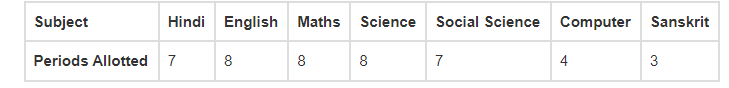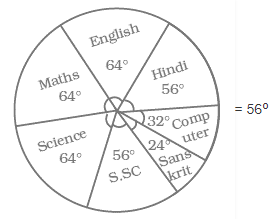# In the time table of a school,

Question:

In the time table of a school, periods allotted per week to different teaching subjects are given below:Draw a pie chart for this data.

Solution:

From the question,

Total number periods allotted = 7 + 8 + 8 + 8 + 7 + 4 + 3 = 45 periods

Central angle of pie chart for each subject,

Hindi = (7/45) × 360English = (8/45) × 360o

= 64o

Maths = (8/45) × 360o

= 64o

Science = (8/45) × 360o

= 64o

Social Science = (7/45) × 360o

= 56o

Computer = (4/45) × 360o

= 32o

Sanskrit = (3/45) × 360o

= 24o